Tamilnadu State Board New Syllabus Samacheer Kalvi 9th Science Guide Pdf Chapter 8 Sound Text Book Back Questions and Answers, Notes.

## Tamilnadu Samacheer Kalvi 9th Science Solutions Chapter 8 Sound

### 9th Science Guide Sound Text Book Back Questions and Answers

Question 1.
Which of the following vibrates when a musical note is produced by the cymbals in a orchestra?
(a) stretched strings
(b) stretched membranes
(c) air columns
(d) metal plates
(d) metal platesQuestion 2.
Sound travels in air:
(a) if there is no moisture in the atmosphere.
(b) if particles of medium travel from one place to another.
(c) if both particles as well as disturbance move from one place to another.
(d) if disturbance moves.
(d) if disturbance moves

Question 3.
A musical instrument is producing continuous note. This note cannot be heard by a person having a normal hearing range. This note must then be passing through
(a) wax
(b) vacuum
(c) water
(d) empty vessel
(b) vacuumQuestion 4.
The maximum speed of vibrations which produces audible sound will be in
(a) seawater
(b) ground glass
(c) dry air
(d) Human blood
(b) ground glass

Question 5.
The sound waves travel faster
(a) in liquids
(b) in gases
(c) in solids
(d) in vacuum
(c) in solids

II. Fill in the blanks.

1. Sound is a ……………….wave and needs a material medium to travel.
longitudinal mechanical2. Number of vibrations produced in one second is………………..
Frequency

3. The velocity of sound in solid is …………… than the velocity of sound in air.
greater

4. Vibration of object produces ……………..
Sound

5. Loudness is proportional to the square of the ……………………
Amplitude of vibration of sound6. …………..is a medical instrument used for listening to sounds produced in the body.
Stethoscope

7. The repeated reflection that results in persistence of sound is called ……………………
ReverberationIII. Match the following :

 1. Tuning fork a. The point where density of air is maximum. 2. Sound b. Maximum displacement from the equilibrium position. 3. Compressions c. The sound whose frequency is greater than 20,000 Hz 4. Amplitude d. Longitudinal wave 5. Ultrasonics e. Production of sound

1 – e, 2 – d, 3 – a, 4 – b, 5 – c

Question 1.
Through which medium sound travels faster, iron or water? Give reason.
Sound travels faster through iron than water.
Reason: The speed of sound depends on the nature of the medium. As Iron is a solid, sound travels faster through iron than Water.

Question 2.
Name the physical quantity whose SI unit is ‘hertz’. Define.
The SI unit of frequency is Hertz. Frequency is the number of waves produced in one second.Question 3.
What is meant by supersonic speed?
Supersonic speed is the speed of an object that exceeds the speed of sound in air (330 ms-1).

Question 4.
How does the sound produced by a vibrating object in a medium reach your ears?

• When an object vibrates, it sets the particles of the medium around it vibrating.
• The vibrating particles in the medium displace from the equilibrium position and exerts pressure on adjacent particles.
• Thus this process continues in the medium till the sound reaches our ear.

Question 5.
You and your friend are on the moon. Will you be able to hear any sound produced by your friend?
We cannot hear any sound on the moon.
Reason: Absence of atmosphere (medium) in the moon.Question 1.
Describe with a diagram, how compressions and rarefactions are produced.
Compressions :
When a vibrating body moves forward, it creates a region of high pressure is known as compressions. In compression, the particles are denser. Compressions are the region where particles are crowded together.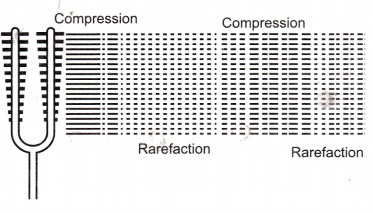Rarefaction :
When the vibrating body moves backward, it creates a region of low pressure (i.e) low density of particles. This is called rarefaction. Rarefactions are the regions where particles are spread apart.

Question 2.
Verify experimentally the reflection of the laws of sound.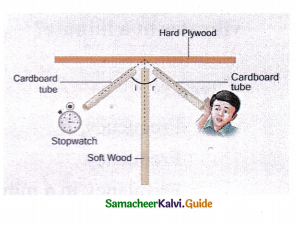• Make two identical long pipes using chart paper.
• Arrange them on a table near wall.
• Keep a clock near the open end of one pipe and hear the sound of the clock through the other pipe.
• Adjust the pipe till the sound of the clock heard with more clarity.
• Now measure the angle of incidence and reflection and see the relationship between the angles.
• The angle in which the sound is incident is equal to the angle in which sound is reflected.
• Direction of incident sound, direction of the reflected and the normal are in the same plane.
• Thus laws of reflection of sound are verified.Question 3.
List the applications of sound.
Applications of ultrasound

• Ultrasound can be used in cleaning technology. Minute foreign particles can be removed from objects placed in a liquid bath through which ultrasound is passed.
• Ultrasounds can also be use d to detect cracks and flaws in metal blocks.
• Ultrasonic waves are made to reflect from various parts of the heart and form the image of the heart. This technique is called ‘echocardiography’.
• Ultrasound may be employed to break small ‘stones’ formed in the kidney into fine grains. These grains later get flushed out with urine.

Question 4.
Explain how do SONAR works?
SONAR stands for Sound Navigation And Ranging. Sonar is a device that uses ultrasonic waves to measure the distance, direction, and speed of underwater objects. Sonar consists of Science – 9 (Physics)
a transmitter and a detector and is installed at the bottom of boats and ships. The transmitter produces and transmits ultrasonic waves. These waves travel through water and after striking the object on the seabed, get reflected back and are sensed by the detector.

The detector converts the ultrasonic waves into electrical signals which are appropriately interpreted. The distance of the object that reflected the sound wave can be calculated by knowing the speed of sound in water and the time interval between transmission and reception of the ultrasound. Sonar technique is used to determine the depth of the sea and to locate underwater hills, valleys, submarines, icebergs etc.VI. Numerical problems :

Question 1.
The frequency of a source of sound is 600 Hz. Calculate the number of times it vibrates in a minute?
Solution :
Given :
Frequency, v = 600 Hz.
Frequency = Number of vibrations in a second.
Frequency in a minute = Fequency per second × 60
= 600 Hz × 60
No. of vibrations in a minute = 36,000 Hz (or) 36 kHz.

Question 2,.
A stone is dropped from the top of a tower 750 m high into a pond of water at the base of the tower. Calculate the number of seconds for the splash to be heard? (Given g = 10 ms-2 and speed of sound = 340 m s-1)
Solution :
Given :
Height of tower, s = 750 m
Acceleration, g =10 ms-2
Speed of sound, v – 340 ms-1
Initial velocity of stone, u =0 (stone at rest)

Consider
(i) Time taken to reach the pond, t1
(ii) Time taken by sound to reach top, t2
According to Equation of Motion, s = ut + $$\frac{1}{2}$$ at2
Here, s = ut1 + $$\frac{1}{2}$$ gt12
Substituting the values, 750 = 0 × t1 + $$\frac{1}{2}$$ × 10 × t12
750 = 5 t12
t12 = 150
t1 = 12.25 s
Time taken by sound to reach top t2 = $$\frac{s}{v}=\frac{750}{340}$$ = 2.2s
t2 = 2.2 s
Time for splash to be heard at top, t = t1 + t2
t = 12.25 + 2.2
t = 14.45 s
Time for splash to be heard at top, t = 14.45 s.Intext Activities

ACTIVITY – 1

Take a tuning fork and strike its prongs on a rubber pad. Bring it near your ear. Do you hear any sound? Now touch the tuning fork with your finger. What do you feel? Do you feel vibrations?

Aim :
To demonstrate the vibration of a molecule.
Materials Required : 1. Tuning fork, 2. Rubber band.

Procedure:

1. Take a tuning fork and strike its prongs on a rubber pad.
2. Bring it near your ear. Hear the sound.
3. Touch the tuning fork with finger.
4. Try to feel the vibrations.

Observation :
When we strike the tuning fork on the rubber pad, it starts vibrating. The vibrations can be felt.

Conclusion :
The tuning fork vibrates the molecules which will vibrate the nearby molecules.
[Vibration = movement of molecule from mean position],

[End of the activity]ACTIVITY – 2

Take a coil or spring and move it forward and backward. What do you observe? You can observe that in some parts of the coil the turns will be closer and m some other parts the turns will be far apart.
Sound also travels in a medium in the same manner. We will study this now.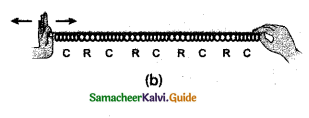Aim :
To demonstrate the propagation of Longitudinal wave. (Sound)
Materials required : 1. Coil, 2. Spring

Procedure:

1. Take a coil or spring
2. Move it forward and backward.

Observations:

1. Some parts of the coil, the turns are closer.
2. In other parts, the turns are far apart.

Conclusion :

1. Closer turns represent compressions.
2. Far apart turns are rarefactions.
3. Longitudinal waves propagate through compressions and rarefactions.

[End of the activity]ACTIVITY – 3

Listen to the audio of any musical instruments like flute, nathaswaram, tabla, drums, veena etc., Tabulate the differences between the sounds produced by the various sources.

Aim :
To compare the sounds of musical instruments.
Materials required: 1. Flute, 2. Drums, 3. Veena,

Procedure :

1. Hear the instrumental music of flute, drums and veena.
2. Tabulate the difference between the sounds.

Observation :

 Name of musical instrument Vibrating Part Characteristics 1. Flute Air column Longer wavelength, low-frequency low pitch. 2. Drums Stretched membrane Shorter wavelength, high-frequency High pitched 3. Veena Stretched string

Conclusion :
Sounds in the different music instruments are characterised by the following qualities such as. 1. Amplitude, 2. The wavelength, 3. Frequency, 4. Pitch, 5. Loudness.

I. Choose the correct answer :

Question 1.
Which of the following is a non-mechanical wave?
(a) sound
(b) water
(c) light
(d) none of the above
(c) lightQuestion 2.
Sound cannot travel through …………………
(a) darkness
(b) gases
(b) vacuum
(d) all of these
(c) vacuum

Question 3.
In longitudinal waves, the particle vibrates in a ………………….. direction of propagation.
(a) parallel
(b) perpendicular
(c) curved
(d) all of these
(b) perpendicularQuestion 4.
Human beings cannot hear …………………
(a) infrasonic sound
(b) sonic sound
(c) ultrasonic sound
(d) a & c
(d) a & c

Question 5.
The frequency greater than 20,000 Hz is ………………. sound.
(a) infrasonic
(b) ultrasonic
(c) audible
(d) sonic
(b) ultrasonic

Question 6.
of sound depends on the amplitude of the wave,
(a) Loudness
(b) Pitch
(c) Timbre
(d) Tone
(a) LoudnessQuestion 7.
………………. is a general term for the distinguishable characteristic of wave
(a) Pitch
(b) Amplitude
(c) Frequency
(d) Timbre
(d) Timbre

Question 8.
Sound travels in air as ……………..wave.
(a) longitudinal
(b) transverse
(c) electromagnetic
(d) all of these
(a) longitudinalQuestion 9.
The distance between two adjacent crest is called …………………..
(a) amplitude
(b) wavelength
(c) time period
(d) frequency
(b) wavelength

Question 10.
When temperature increases the speed of sound
(a) increases
(b) decreases
(c) remains same
(d) none of these
(a) increases

Question 11.
The relation between speed, frequency and wavelength is ………………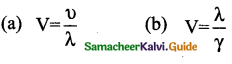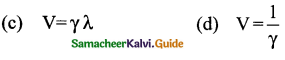(c) V = γλQuestion 12.
SI unit of wavelength is ……………………………..
(a) Hertz
(b) metre per second
(c) decibel
(d) metre
(d) metre

Question 13.
The speed of sound in a gaseous medium depends on …………….
(a) pressure
(b) temperature
(c) density
(d) all of these
(d) all of these

Question 14.
The elastic property of medium is a factor of …………. medium.
(a) gas
(b) liquid
(c) solid
(d) none of these
(c) solidQuestion 15.
To hear an echo, the minimum distance of the obstacle from the source of sound must be ………………
(a) 34 m
(b) 17 m
(c) 340 m
(d) 170 m
(b) 17 m

Question 16.
The part of ear that turns pressure variations into electrical signals is .
(a) the hammer
(b) anvil
(c) cochlea
(d) stirrup
(c) cochlea

Question 17.
The frequency of source of sound is 60 Hz. Then the number of vibrations in a minute will be ………………..
(a) 36
(b) 360
(c) 3600
(d) 6000
(c) 3600

Question 18.
Sonar is a device that uses to measure the distance, direction and speed of the underwater objects.
(b) infrared waves
(c) water waves
(d) ultrasonic waves
(d) ultrasonic wavesQuestion 19.
The speeds of sound in four different media are given below. Which of the following is the most likely speed in ms-1 with which the two underwater whales in a sea can talk to each other when separated by a large distance?
(a) 5170
(b) 1280
(c) 340
(d) 1530
(d) 1530

[Note = Speed of sound in Sea Water is 1530 ms-1]

II. Fill in the blanks :

1. The propagating disturbance that travels in a medium is called a ………………
Wave

2. Rarefactions are the regions of ……………. where particles are spread apart.
low pressure

3. Sound travels faster in ……………. and slower in ……………..
Solid, Gas

4. The loudness of sound depends on the ……………. of the sound wave.
Intensity

5. A sound of single frequency is called …………….and a collection of tones is called .
Tone, Note6. The speed of sound in air at 0°C is ……………..
330 ms-1

7. …………….is an unwanted sound.
Noise8. Loudness equal to (or) greater than …………….can be painful to the ear.
120 dB

9. …………….allows bats to navigate through dark caves and find insects for food.
Echolocation

10. …………….is an image obtained by the use of reflected ultrasonic waves.
An echogram

11. Depth of an ocean can be determined by …………….
SONAR12. The distance of underwater objects can be deduced by the formula …………….
Id = v x t

13. …………….muscle contracts in response to electrical depolarisation of the muscle cells.
Cardiac

14. Velocity of sound by a tuning fork frequency of 480 Hz is 340 ms Its wavelength is …………….
0.7 m

15. The part of ear that collects the sound from the surroundings is . …………….
Pinna

16. Ultrasounds can also be used to detect cracks and flaws in …………….
Metal blocks17. In the inner ear, the pressure variations are turned into electrical signals by the …………….
Cochlea

III. Match the following :

I.

 1. Stethoscope a) Repeated reflections 2. Echogram b) Multiple reflections 3. SONAR c) Ultrasonic waves 4. Reverberation d) Speed of underwater objects

1-b, 2-c,3-d,4-a

II.

 1.  Wave a) Unwanted sound 2. Sound b) Successive reflections 3. Noise c) Movement of disturbance 4. Thunder d) Mechanical wave

1-c, 2-d, 3 -a, 4-bIII.

 1. Cochlea a) Amplification of vibrations 2. Pinna b) Electrical signals to the brain 3. Ear bones c) Pressure variations to an electrical signal 4. Auditory nerve d) Collection of sound

1-c, 2-d,3 -a,4-bIV. Assertion and Reason type questions :

Mark the correct choice as :
(a) If both Assertion and Reason are true and Reason is the correct explanation of
Assertion. .
(b) If both assertion and reason are true but reason is not the correct explanation of assertion.
(c) If assertion is true but reason is false.
(d) If assertion is false but reason is true.

Question 1.
Assertion : Human beings Cannot hear infrasonic sound.
Reason : Sound with frequency less than 20 Hz is called infrasonic sound.
(a) Both Assertion and Reason are true and Reason . is the correct explanation of AssertionQuestion 2.
Assertion : As the amplitude of sound increases, the loudness of sound decreases.
Reason : The loudness of sound depends on the amplitude of the wave.
(d) Assertion is false but reason is true
Correct Assertion : As the amplitude of sound decreases, the loudness of sound decreases.

Question 3.
Assertion : The sound of thunder is heard a little latter than the flash of light.
Reason : The speed of sound is much less than the speed of light.
(a) Both Assertion and reason are true and Reason is the correct explanation of AssertionQuestion 4.
Assertion : The speed of sound in air is less than speed of sound in solid.
Reason : If we increase the temperature of medium, the speed of sound increases in any medium.
(b) Both assertion and reason are true but reason is not the correct explanation of assertion
Reason : The particles in sound are closer in solids than air.

Question 5.
Assertion : The sound which we hear again is called Echo.
Reason : The sensation of sound (Echo) persists in our brain for about 1 s.
(c) Assertion is true but reason is false
Reason : The sensation of sound persists in our brain for about 0 – 1 s.

V. State whether true or false. If false, correct the statement:

1. Vibrating particles travel all the way from vibrating object to ear. ,
False.
Correct statement: Vibrating particles do not travel all the way from vibrating object to ear.2. The small to and fro motion is called vibration.
True.

3. Pitch is a general term for the distinguishable characteristic of a tone.
false.
Correct statement: Timbre is general term for the distinguishable characteristic of a tone.

4. The speed of sound in a gaseous medium depends on the nature of gas.
True.

5. The direction of incident sound, direction of reflected sound and the normal are in different Planes.
False.
Correct statement: The direction of incident sound, direction of reflected sound and the normal are in same plane.

6. Ultrasonic sounds cannot be heard by the Human beings.
True.7. The Eardrum moves inward when a rarefaction reaches it.
False.
Correct statement: The Eardfum moves outward when a rarefaction reaches it.

VI. Very Short Answer questions :

Question 1.
What is a wave?
The disturbance which is carried forward in a medium is called wave.

Question 2.
Distinguish between compression and rarefactions.
Compression :
Compressions are the regions where particles are crowded together.

Rarefaction :

Rarefactions are the regions of low pressure where particles are spread apart.

Question 3.
Name the five characteristics of’a sound wave. ‘
1. Amplitude, 2. Frequency, 3. Time period, 4. Wavelength, 5. Velocity or speed.

Question 4.
Name the distinguishing factors of sound.
1. Loudness, 2. Pitch, 3. Timbre (or) quality.

Question 5.
Define : Intensity.
Intensity is defined as the amount of energy per unit time perpendicular to the direction of propagation of the wave.

Question 6.
Differentiate tone from note.
Tone : A sound of single frequency
Note : A collection of tones.Question 7.
What is Reverberation?
The repeated reflection that results in persistent sound is called reverberation.

Question 8.
Expand the abbreviation of the term SONAR.
SONAR – SOUND NAVIGATION AND RANGING.

Question 9.
What type of waves are illustrated by the movement of a rope whose one end is moved up and down?

• The movement of rope whose one end is moved up and down is an example of
Transverse wave.
• The movement of particles of the medium is perpendicular to the direction of
propagation of the wave is called Transverse Wave.

Question 10.
Name the type of waves produced when a tuning fork is struck in air.
Longitudinal waves are produced when a tuning fork is struck in air.Question 11.
A stone is dropped on the surface of water in a pond. Name the types of waves produced.
Transverse waves are produced when a stone is dropped into the pond.

Question 12.
State one observation from every day life which shows that sound travels much more slower than light.
The flash of lightening is seen before we hear the sound of thunderstorm.
Reason : The speed of sound (344 m/s) is less than the speed of light (3 × 108 m/s)

Question 13.
What name is given to those air-crafts which fly at speeds greater than the speed of sound?
Supersonic Aircraft.

Question 14.
Name the device which is used to produce sound in laboratory experiments.
Tuning fork is used to produce sound in laboratory experiments.

Question 15.
What should an object do to produce sound?

• An object should vibrate in a medium.
• Vibrations travel as disturbances to reach our ears as sound.Question 16.
Can sound travel through vacuum?

• Sound cannot travel through vacuum.
• Sound needs a material medium to travel like air, water, steel, etc.

Question 1.
How do we hear sound?

• Mechanical energy vibrates an object.
• The vibrations reach our ear and we hear sound.

Question 2.
Why are sound waves called mechanical waves?
Sound waves are called as mechanical waves because sound waves need a medium to propagate and propagation through medium due to interaction of the particles.

Question 3.
Define : (i) Amplitude (ii) Frequency
Amplitude (A) : The maximum displacement of the particles of the medium from their original undisturbed position when a wave passes through the medium. Unit: metre (m).
Frequency (n) : The number of vibrations produced in one second is called Frequency of the wave. Unit: Hertz (Hz) or s-1

Question 4.
Define : Wavelength (λ) and Timbre
Wavelength (λ) : The maximum distance in which a sound wave repeats itself (or) In a Sound Wave the distance between the centres of two consecutive compressions (or) two consecutive rarefactions. Unit: metre (m).
Timbre: Timbre is the characteristic which distinguish two sounds of same loudness and pitch of two different instruments.Question 5.
How are the Wavelength and frequency of a Sound wave related to its speed?
Speed (v), Wavelength (λ) and frequency of sound (υ) are related by the following equation: Speed (v) = Wavelength (λ) × Frequency (υ) => v = λ × υ

Question 6.
What are the factors that intensity of Sound depends upon?
The intensity of sound depends on the

• Amplitude of the source.
• Distance of the observer from the source.
• Surface area of the source.
• Density of the medium.
• Frequency of the source.

Question 7.
On which factors the speed of sound in gaseous medium depends?

• Pressure of the medium.
• Temperature of the medium.
• Density of the medium.
• Nature of the medium.Question 8.
On which factors the speed of sound in solid medium depends?

• Elastic property of the medium.
• Temperature of the medium.
• Density of the medium.

Question 9.
Mention the fequency ranges of infrasoncis and ultrasonics.

• Sound waves with frequencies below audible range is termed as Infrasonics.
Infrasonics: Frequencies less than 20 Hz.
• Sound waves with frequencies above audible range is called as Ultrasonics.
Ultrasonics: Frequencies greater than 20,000 Hz.

Question 10.
Why is a sound wave called a longitudinal wave?
Sound wave is called a Longitudinal wave because

• It produces compressions and rarefactions in the air.
• The particles of air vibrates parallel to the direction of propagation.Question 11.
Which characteristics of the sound helps you to identify your friend by his voice while sitting with others in a dark room?
The Timbre (or) quality of sound enables us to identify our friend by his voice.

Question 12.
List the causes of noise pollution.

• Hearing problems.
• Rupture of eardrum and hearing loss.
• Psychological damages.

Question 13.
Why are roots and walls of the auditorium covered with rough plaster?
To reduce the effect of reverberation in large halls of the auditorium.

Question 14.
Give two practical applications of reflection of Sound?

• SONAR – Reflection of sound is used to measure the distance, direction and speed of underwater objects.
• Stethoscope – Patient’s heartbeats reach doctor’s ear by multi reflections of sound.Question 15.
What is acoustics?
Acoustics is a branch of physics that deals with the study of properties of Sound.

Question 1.
Prove that sound needs a material medium for its propagation.
To prove sound needs a material medium.

• Take an electric bell and an airtight glass jar connected to a vacuum pump.
• Suspend the electric bell inside the airtight jar and press the switch of the bell and hear the sound of the bell.
• Now pump out the air from the glass jar gradually.
• If the jar has vaccum, any sound will not be heard.
• So, sound needs a material medium to travel (here, it is air) and it is proved.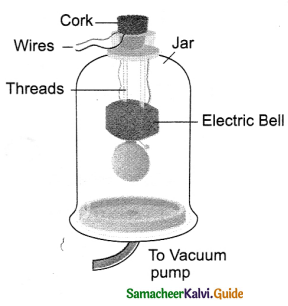Question 2.
Distinguish between Loudness and Intensity of the Sound?
Loudness

1. Loudness is a quantity by virtue of which sound can be distinguished.
2. The loudness of the sound is determined by its amplitude. Loudness a (Amplitude) .

Intensity

1. Intensity of the sound wave is defined as the amount of sound energy passing through unit area per second.
2. The amplitude of sound decides the intensity.

Question 3.
What is an echo? Write the conditions to hear an echo.
A repeated sound that is caused by the reflection of sound waves from a surface.

Conditions for echo

• For echo, the time interval between the original sound and reflected sound must be 0.1 s. The sound must go to the obstacle and return to the listener on reflection after 0.1s.
• Total distance covered by the sound from source to the obstacle and reflected to listener must be atleast 340 ms-1 × 0.1s = 34m.
• So the minimum distance of the obstacle from the source of sound = half of total distance
$$\frac{34 \mathrm{~m}}{2}$$ = 17m.
• This distance will change with the temperature of air.Question 4.
Explain the working of human ear with diagram.
Working of Human Ear
Parts of human ear are (i) outer ear, (ii) middle ear, (iii) inner ear.
(i) Outer ear [Pinna] : It collects the sound from the surroundings and directs towards auditory canal.
(ii) Middle ear : Sound reaches the auditory canal and vibrates the eardrum (or) tympanic membrane, a thin membrane.
Three small bones (hammer, anvil, stirrup) amplify the vibrations, several times. The middle ear transmits the amplified pressure variations to the inner ear.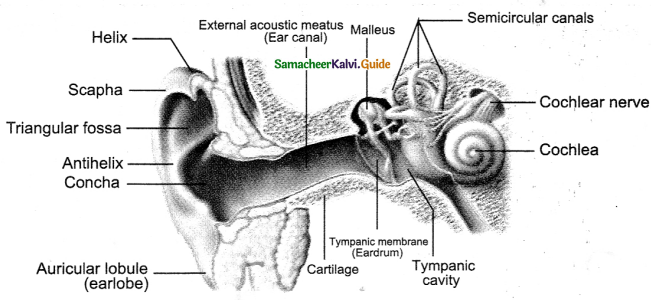(iii) Inner ear : (Cochlea) (j) Receives vibrations, (ii) converts them into electrical signals and (iii) they are sent to the Brain thro’ auditory nerve.
The Brain interprets the electrical signals to sound.

IX. Numerical problems :

Question 1.
The frequency of tuning fork is 484 Hz. What is the time period?
Solution :
Frequency of tuning fork, n = 484 Hz.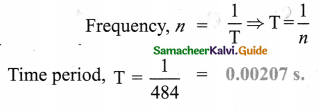Question 2.
Velolcity of sound in air is 344 ms-1 What is the range of wavelength of audible sound?
Solution :
Velocity of sound in air, v = 344 ms-1
Audible frequency range = 20 – 20,000 Hz
We know V = $$v \lambda \Rightarrow \lambda=\frac{v}{v}$$
When Fequency v = 20Hz
$$\lambda=\frac{344}{20}=17.2 \mathrm{~m}$$
When Frequency, v = 20,000Hz
$$\lambda=\frac{344}{20,000}=0.0172 \mathrm{~m}$$
Range of wavelength of audible Sound = 0.0172 m- 17.2 m

Question 3.
A body vibrates 3000 times in one minute. If the velocity of sound in air 330 ms-1, find (i) Frequency of vibration (ii) Wavelength.
Solution :
Velocity of sound in air, v = 330 ms-1
No.of vibrations in a minute = 3000 / minute.
(i) Frequency, (Vibrations produced in one second)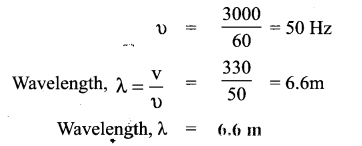Question 4.
A person is listening to a tone of 400 Hz from a distance of 500 m from the source of sound. What is the time interval between successive compressions from source?
Solution :
Fequency of tone u = 400 Hz.
Time interval between two
successive compressions
from source = Time period of wave.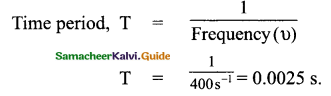Time interval between two successive compressions from source = 0.0025 s.Question 5.
An echo returned in 3s. What is the distance of reflecting surface from the source given that the speed of sound is 330 ms-1.
Solution :
Speed of sound, v = 330 ms-1
Time of the echo, t = 3s.
Total distance travelled by the sound 2d = v × t = 330 × 3
2d = 990 m.
2d = Distance from source to reflecting surface + distance from reflecting surface to the listener.
So, distance from the source to reflected surface = $$\frac{\text { Total distance }}{2}$$
= $$\frac{2 d}{2}$$
Distance of the reflecting surface from the source = $$\frac{990}{2}$$
= 495m

Question 6.
Two persons are at opposite ends of a steel rod. One strikes the end of rod with a stone. Find the ratio of time taken by the sound wave in air and in steel to reach the second person. (Sound of speed in steel = 5960 ms-1, Sound of speed in air = 344 ms-1)
Solution :
Sound of speed in steel = 5960ms-1
Sound of speed in air =344ms-1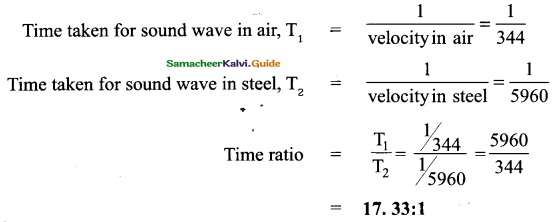Question 7.
A Sound wave travels at a speed of 344 ms-1. If the wavelength is 1.5 cm, what is the frequency of Wave? Will it be audible?
Solution :
Velocity of soung , v = 344ms-1
Wavelength of sound. λ = 1.5cm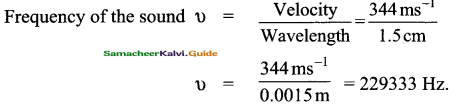Audible frequency range = 20 – 20000Hz
So frequency 2,29,333 is not audible.